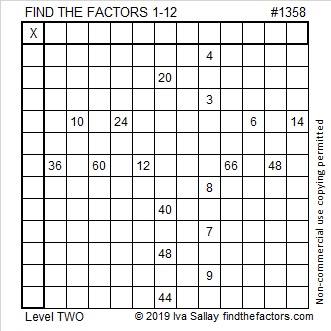# 1358 and Level 2

If you are familiar with a basic 12 × 12 multiplication table, then you can solve this puzzle. The clues aren’t in the same order as they are in the table, but that only makes it a little more challenging.Print the puzzles or type the solution in this excel file: 12 Factors 1357-1365

Here’s a little bit about the number 1358:

• 1358 is a composite number.
• Prime factorization: 1358 = 2 × 7 × 97
• 1358 has no exponents greater than 1 in its prime factorization, so √1358 cannot be simplified.
• The exponents in the prime factorization are 1, 1, and 1. Adding one to each exponent and multiplying we get (1 + 1)(1 + 1)(1 + 1) = 2 × 2 × 2 = 8. Therefore 1358 has exactly 8 factors.
• The factors of 1358 are outlined with their factor pair partners in the graphic below.910-1008-1358 which is 14 times (65-72-97)

OEIS.org informs us that 1358!!!! + 1 is a prime number.

This site uses Akismet to reduce spam. Learn how your comment data is processed.